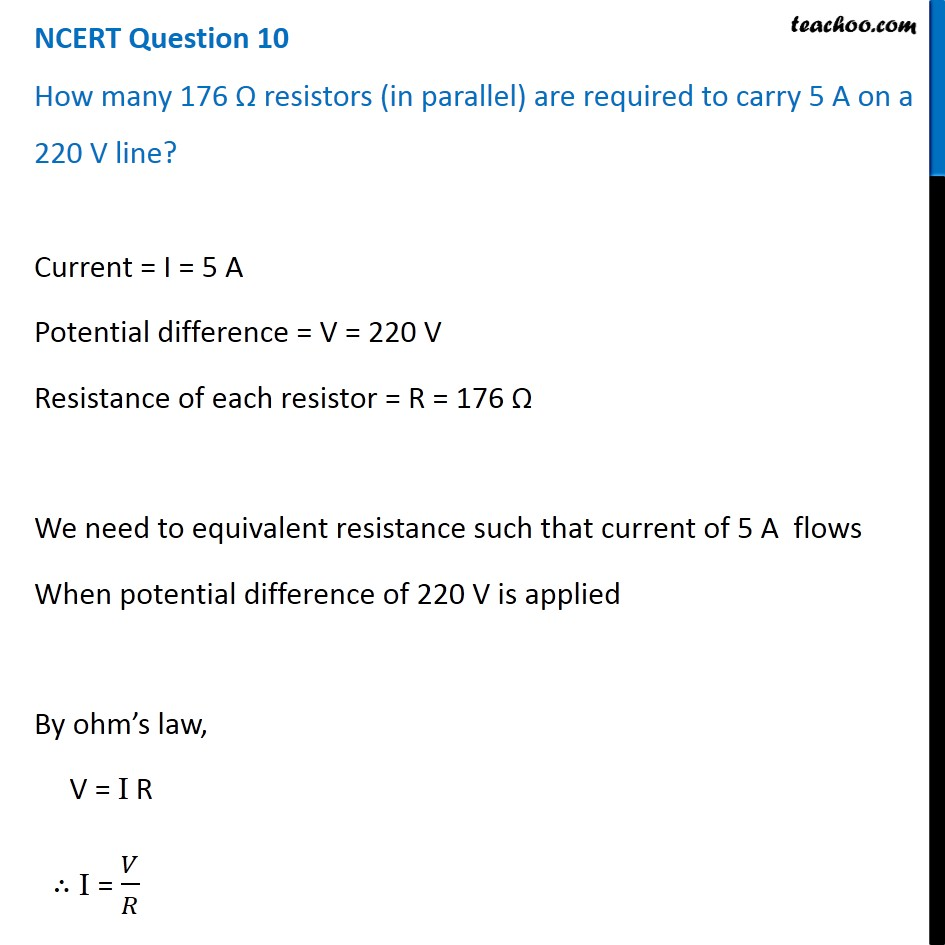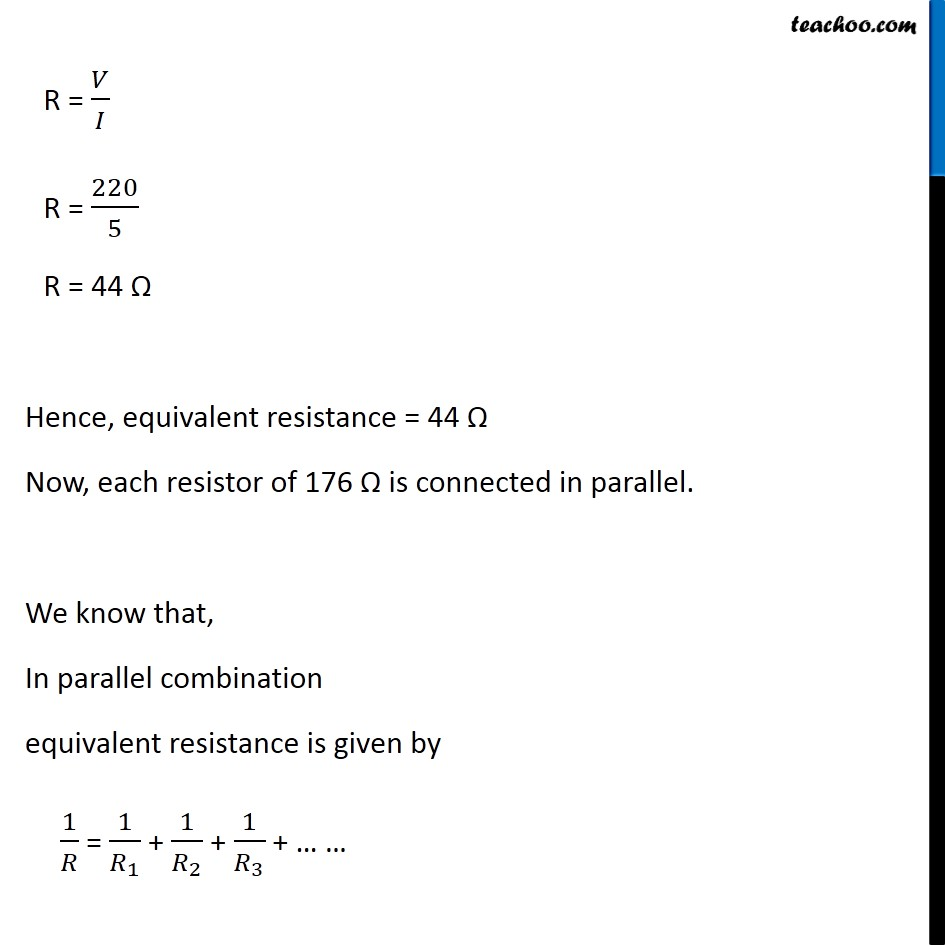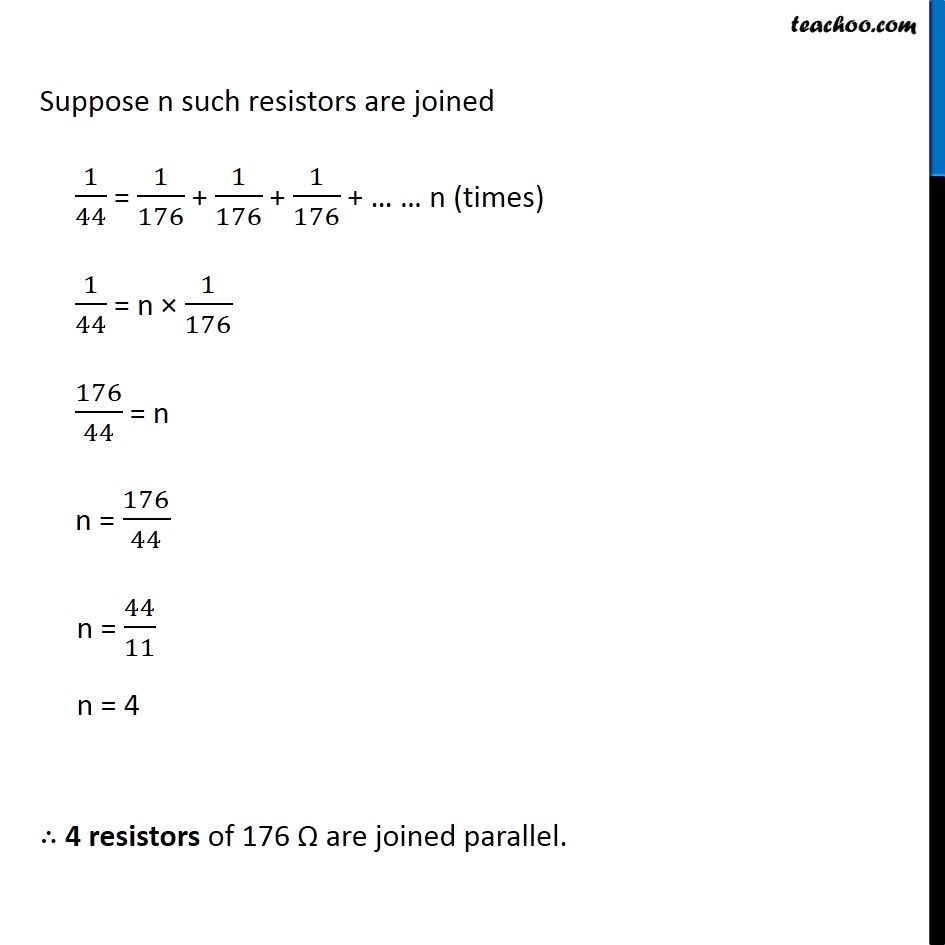NCERT Questions

Class 10
Chapter 12 Class 10 - Electricity

## How many 176 Ω resistors (in parallel) are required to carry 5 A on a 220 V line?Learn in your speed, with individual attention - Teachoo Maths 1-on-1 Class

### Transcript

NCERT Question 10 How many 176 Ω resistors (in parallel) are required to carry 5 A on a 220 V line? Current = I = 5 A Potential difference = V = 220 V Resistance of each resistor = R = 176 Ω We need to equivalent resistance such that current of 5 A flows When potential difference of 220 V is applied By ohm’s law, V = I R ∴ I = 𝑉/𝑅 R = 𝑉/𝐼 R = 220/5 R = 44 Ω Hence, equivalent resistance = 44 Ω Now, each resistor of 176 Ω is connected in parallel. We know that, In parallel combination equivalent resistance is given by 1/𝑅 = 1/𝑅_1 + 1/𝑅_2 + 1/𝑅_3 + … … Suppose n such resistors are joined 1/44 = 1/176 + 1/176 + 1/176 + … … n (times) 1/44 = n × 1/176 176/44 = n n = 176/44 n = 44/11 n = 4 ∴ 4 resistors of 176 Ω are joined parallel.